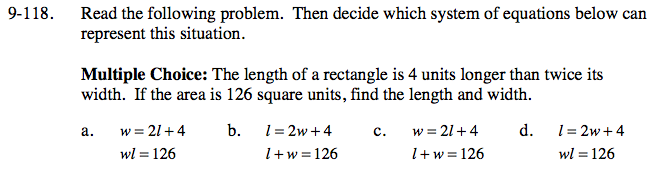### Home > CCA > Chapter 9 > Lesson 9.4.3 > Problem9-118

9-118.
1. Read the following problem. Then decide which system of equations below can represent this situation. Homework Help ✎

2. Multiple Choice: The length of a rectangle is 4 units longer than twice its width. If the area is 126 square units, find the length and width.

1. w = 2l + 4
wl = 126

2. l = 2w + 4
l + w = 126

3. w = 2l + 4
l + w = 126

4. l = 2w + 4
wl = 126Write the equations based on the information given above. Choose the letter that exactly represents your answer.